Courses

# Doc: Work and Power Class 11 Notes | EduRev

## Class 11 : Doc: Work and Power Class 11 Notes | EduRev

The document Doc: Work and Power Class 11 Notes | EduRev is a part of the Class 11 Course Physics For JEE.
All you need of Class 11 at this link: Class 11

Introduction

• Work is said to be done by a force when the force produces a displacement in the body on which it acts in any direction except perpendicular to the direction of the force.
• Work and energy are closely related to each other. Work can also be defined as the transfer of energy. In Physics, for two objects, the work done is defined as the transfer of energy from the first object to the second object. Also, energy is defined as the capacity to do work.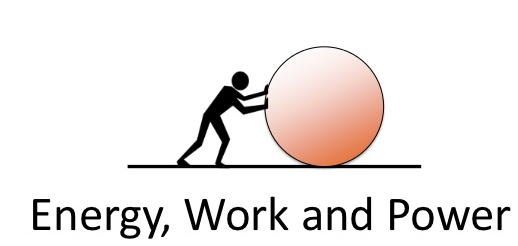• Work is believed to be done by a force when an object experiences displacement parallels to the line of action of the force. It’s an activity that includes force and movement in the direction of the force. The capability for doing work is what the energy is! Let’s learn more about this.
Work Done by a Constant Force
• Consider an object undergoes a displacement S along a straight line while acted on a force F that makes an angle q with S as shown.
• The work done W by the agent is the product of the component of force in the direction of displacement and the magnitude of displacement.
i.e., W = FS cosθ ...(1)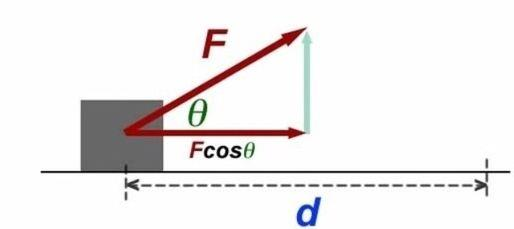• Work done is a scalar quantity and its S.I. unit is N-m or joule (J). We can also write work done as a scalar product of force and displacement.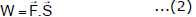where S is the displacement of the point of application of the force
From this definition, we conclude the following points

➢ Work done by a force is zero if displacement is perpendicular to the force (q = 90°)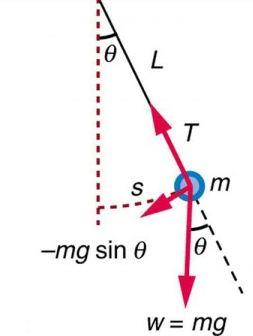Example.

The tension in the string of a simple pendulum is always perpendicular to displacement. (Figure)

So, work done by the tension is zero.

If the angle between force and displacement is acute (q < 90°), we say that the work done by the force is positive.

Example:

When a load is lifted, the lifting force and the displacement act in the same direction. So, work done by the lifting force is positive.

Example:

When a spring is stretched, both the stretching force and the displacement act in the same direction. So work done by the stretching force is positive.

➢ If the angle between force and displacement is obtuse (q > 90°), we say that the work done by the force is negative.

Example:

When a body is lifted, the work done by the gravitational force is negative. This is because the gravitational force acts vertically downwards while the displacement is in the vertically upward direction.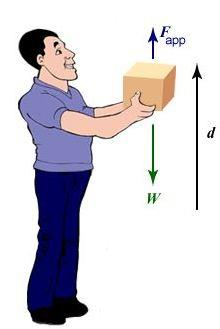Fig: Forces acting on a box.

• Work is said to be done by a force when its point of application moves by some distance.Force does no work if point of application of force does not move (S = 0)
Example:
• A person carrying a load on his head and standing at a given place does no work.
• Work is defined for an interval or displacement. There is no term like instantaneous work similar to instantaneous velocity.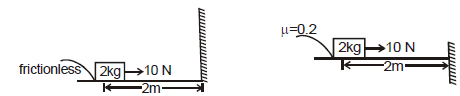• Work done by 10 N force in both the cases are same = 20 N.
• For a particular displacement, work done by a force is independent of type of motion i.e. whether it moves with constant velocity, constant acceleration or retardation etc.
• If a body is in dynamic equilibrium under the action of certain forces, then total work done on the body is zero but work done by individual forces may not be zero.
• When several forces act, work done by a force for a particular displacement is independent of other forces.
• A force is independent of reference frame. Its displacement depends on frame so work done by a force is frame dependent therefore work done by a force can be different in different reference frame.
Units of Work

In cgs system, the unit of work is erg.

One erg of work is said to be done when a force of one dyne displaces a body through one centimetre in its own direction.

1 erg = 1 dyne × 1 cm = 1 g cm s-2 × 1 cm = 1 g cm2 s-2

Note : Another name for joule is newton metre.

Relation between joule and erg:

1 joule = 1 newton × 1 metre

1 joule = 105 dyne × 102 cm = 107 dyne cm

1 joule = 107 erg

1 erg = 10-7 joule

➢ Dimensions of Work

[Work] = [Force] [Distance] = [MLT-2] [L] = [ML2T-2]

Work has one dimension in mass, two dimensions in length and `-2' dimensions in time, On the basis of dimensional formula, the unit of work is kg m2 s-2.

Note that 1 kg m2 s-2 = (1 kg m s-2) m = 1 N m = 1 J.

Work Done by Multiple Forces

If several forces act on a particle, then we can replace in equation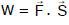by the net force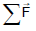where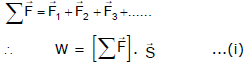This gives the work done by the net force during a displacement of the particle.

We can rewrite equation (i) as :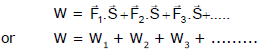So, the work done on the particle is the sum of the individual work done by all the forces acting on the particle.

Q.1.  A block of mass M is pulled along a horizontal surface by applying a force at an angle q with horizontal. Coefficient of friction between block and surface is m. If the block travels with uniform velocity, find the work done by this applied force during a displacement d of the block.

Ans: The forces acting on the block are shown in Figure. As the block moves with uniform velocity the resultant force on it is zero.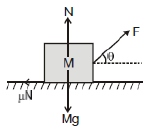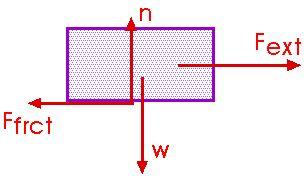F cos θ = μN    ...(i)
F sin θ + N = Mg    ...(ii)
Eliminating N from equations (i) and (ii),

F cos θ = n(Mg - Fsin θ)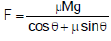Work done by this force during a displacement d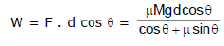Q.2. A particle moving in the xy plane undergoes a displacement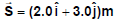while a constant force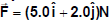acts on the particle.

(a) Calculate the magnitude of the displacement and that of the force.

(b) Calculate the work done by the force.

Ans: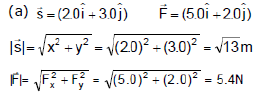(b) Work done by force,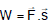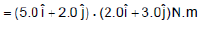= 10 + 0 + 0 + 6=16 N.m = 16 J

Q.3. A block of mass m is placed on an inclined plane which is moving with constant velocity v in horizontal direction as shown in figure. Then find out work done by the friction in time t if the block is at rest with respect to the incline plane.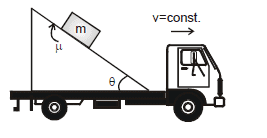Ans: F.B.D of block with respect to ground.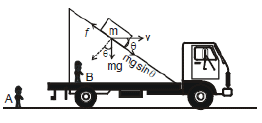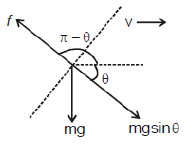Block is at rest with respect to wedge

⇒ f = mg sinθ

In time t the displacement of block with respect to ground d = vt

Work done by friction for man A

Wf = (component of friction force along displacement) × displacement

Wf = mgsinθ.vt cos(180°- θ)

Wf = - mg vt cosθ sinθ

Wf for man B = 0 (displacement is zero with respect to man B)

Work Done by a Variable Force

➢ When F as a function of x, y, z

• When the magnitude and direction of a force vary in three dimensions, it can be expressed as a function of the position.
• For a variable force work is calculated for infinitely small displacement and for this displacement force is assumed to be constant.
dW =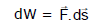• The total work done will be sum of infinitely small work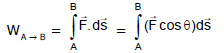• It terms of rectangular components,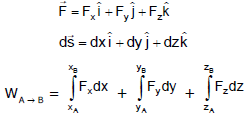Q.4. A force F = (4.0 x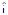+ 3.0 y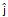) N acts on a particle which moves in the x-direction from the origin to x = 5.0 m. Find the work done on the object by the force.

Ans. Here the work done is only due to x component of force because displacement is along x-axis.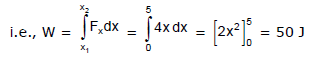Q.5. A force F = 0.5x + 10 acts on a particle. Here F is in newton and x is in metre. Calculate the work done by the force during the displacement of the particle from x = 0 to x = 2 metre.

Ans. Small amount of work done dW in giving a small displacement is given by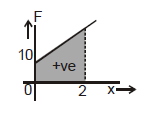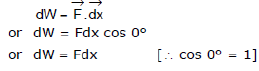Total work done, W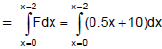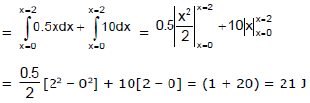➢ When F is given as a function of Time(t)

Q.6. The force F = 2t2 is applied on the 2 kg block. Then find out the work done by this force in 2 sec. Initially at time t = 0, block is at rest.

Ans.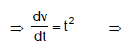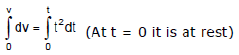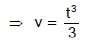Let the displacement of the block be dx from t = t to t = t +dt then, work done by the force F in this time interval dt is.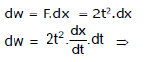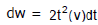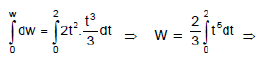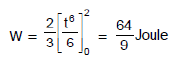➢ Area Under Force Displacement Curve

• Graphically area under the force-displacement is the work done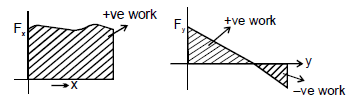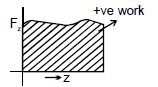• The work done can be positive or negative as per the area above the x-axis or below the x-axis respectively.

Q.7. Force acting on a particle varies with x as shown in figure. Calculate the work done by the force as the particle moves from x = 0 to x = 6.0 m.

Ans: The work done by the force is equal to the area under the curve from x = 0 to x = 6.0 m.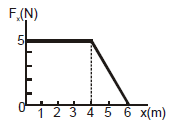This area is equal to the area of the rectangular section from x = 0 to x = 4.0 m plus the area of the triangular section from x = 4.0 m tox = 6.0 m. The area of the rectangle is (4.0) (5.0) N.m = 20 J, and the area of the triangle is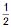(2.0), (5.0) N.m = 5.0 J. Therefore, the total work done is 25 J.

Offer running on EduRev: Apply code STAYHOME200 to get INR 200 off on our premium plan EduRev Infinity!

## Physics For JEE

188 videos|517 docs|265 tests

,

,

,

,

,

,

,

,

,

,

,

,

,

,

,

,

,

,

,

,

,

;